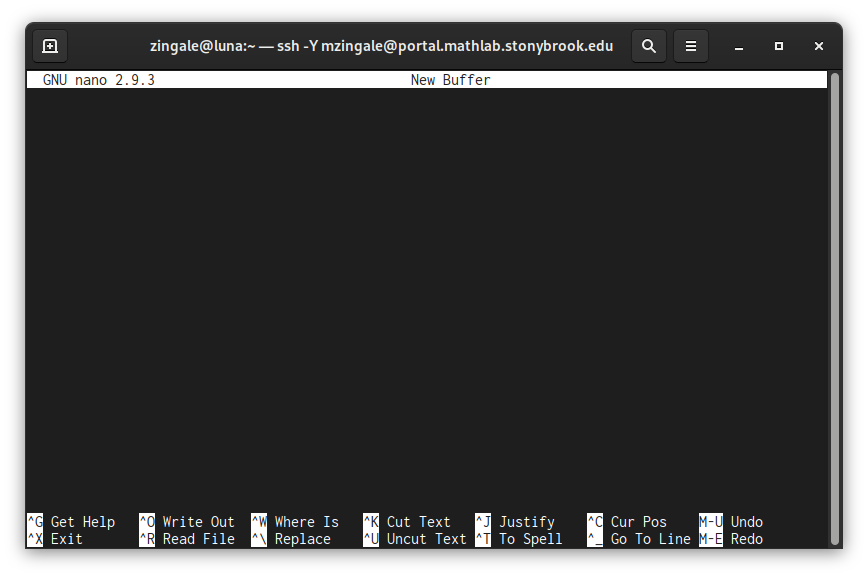# Hello, World¶

Just to make sure we have the necessary tools for this course, we’ll do a quick “Hello, World!” program.

This will use a few concepts that we will explore in depth shortly.

To write the code for our program, we need an editor. We’ll use the simple nano editor here, but we’ll explore others in a bit.

We start at the Unix command line, indicated by the $ prompt. Open nano on the command line simply by typing nano:  span.prompt1:before { content: "$ ";
}
nano


You should have a window that looks something like this:Now we can simply type our C++ code in the window. Enter the following:

#include <iostream>

int main() {
std::cout << "Hello, World!" << std::endl;
return 0;
}


Now save it by doing ^O, where ^ here means you hold down the control key on the keyword.

It will ask for a name, so we’ll use hello.cpp.

Now we can exit via ^X.

We just created the source code for our first program. We now need to compile it into instructions the computer understands. The C++ compiler we will be using is called g++ and we compile this simple program as:

g++ -o hello hello.cpp


Then we can run our program as:

./hello


## Next Steps¶

There is quite a lot going on here that we need to understand before we dive more deeply into C++:

• We started out with a simple \$ prompt in our terminal. What else can we do there?

• How does Unix manage files (like hello.cpp) and how can we copy, modify, move, etc. them?

• What is that compiler command doing? what does the -o mean?

• What is the meaning of the ./ that we put in front of hello?

We’ll start by learning more about Unix, the Unix filesystem, and how to work with the command line.

After that’ we’ll move onto programming.